Typical Transfer Functions
and their corresponding Frequency Domain Plots

1. First order lag: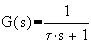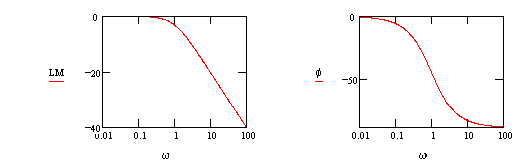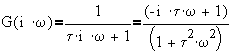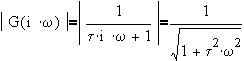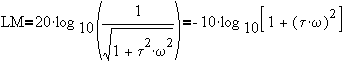@ low frequency,     LM=0
@ high frequency,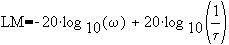which is a line whose slope is -20 db/decade and an "x-intercept" at w=1/t. @ w=1/t,    LM=-10 log10( 2 )= -3.01 dB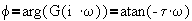@ low frequency,     f=0   @ high frequency,     f= -90o   @ w=1/t,     f= -45o    slope= -66o/decade
2. First order lead:    G(s) = t s + 1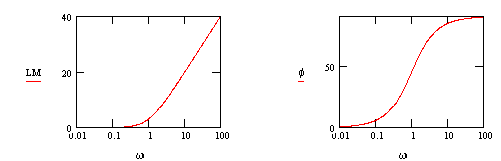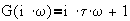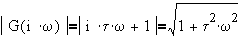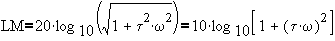@ low frequency,    LM = 0

@ high frequency,    LM=20 log10( w ) + 20 log10( t )

which is a line whose slope is +20 db/decade and an "x-intercept" at w=1/t. @ w=1/t,  LM=10 log10( 2 )= 3.01 dB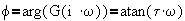@ low frequency,    f=0o

@ high frequency,    f=90o

3. Second Order Underdamped Lag: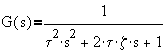(z<1)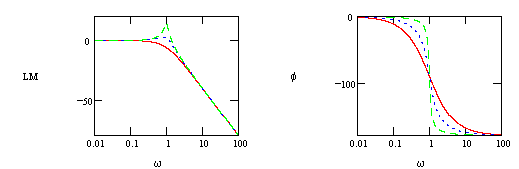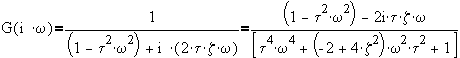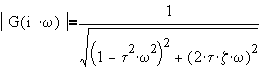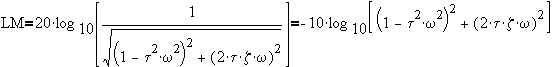@ low frequency,    LM=0
@ high frequency,which is a line whose slope is +40 db/decade and an "x-intercept" at w=1/t.
To determine the peak, take derivative of LM with respect to w and then set to zero.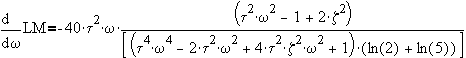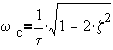®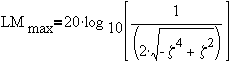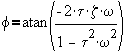@ low frequency,     f=0o
@ high frequency,     f=180o
@ w=1/t,        f=180o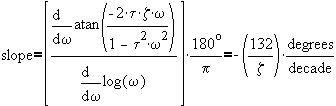4. Delay: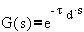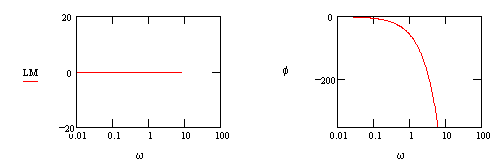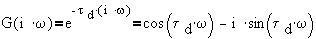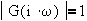LM=0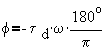@    w=1/td f = -57.3o
@ w=2/td f = -114.6o
@ w=3/td f = -171.9o

5. Integrator: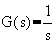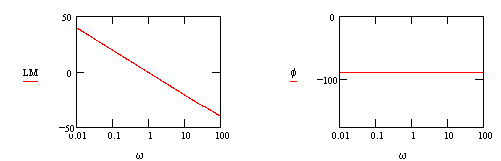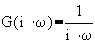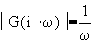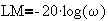: line with a slope = - 20dB/decade
@ w=1     LM=0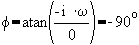5. Differentiator:        G(s) = s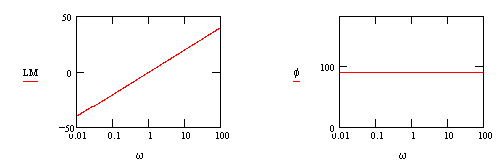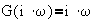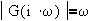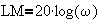: line with a slope = + 20dB/decade

@ w=1     LM=0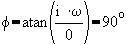Frequency Plots of Higher Order Transfer Functions

Let    G(s)= G1(s) G2(s)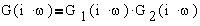In polar form,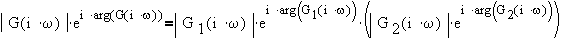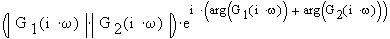thus,
1)  arg(G) = arg(G1) + arg(G2)    or    f = f1 + f2
2)    | G | = | G1 | | G2 |
In terms of log modulus,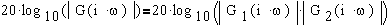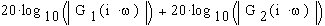or      LM( G ) = LM( G1 ) + LM( G2 )

Implications:

1)    Putting a constant gain K in series with G means | KG | = | K | | G | and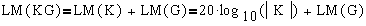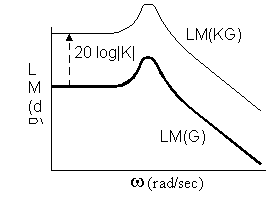Log modulus plot is translated vertically upward if | K | > 1 (downward if | K | < 1)
Since arg(K)=0, the phase shift vs frequency plot remains unchanged.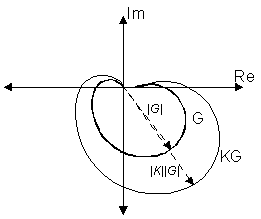The Nyquist plot expands proportionally away from origin if |K| > 1 ( and shrinks if |K| < 1)
2.     At high frequencies, every polynomial order in the denominator contributes -90o in the phase shift plot; while every polynomial order in the numerator contributes +90o.

3.     Using rough line segments for approximate plots, various lead and lags can be combined to produce desired "reshaping" of transfer functions.

Example:

The "real" PID has the transfer functionAdding the following log modulus plots: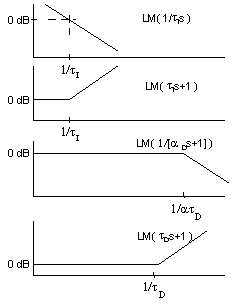yields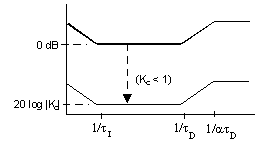This page is maintained by Tomas B. Co (tbco@mtu.edu). Last revised 2/10/1999.
Tomas B. Co
Associate Professor
Department of Chemical Engineering
Michigan Technological University
1400 Townsend Avenue
Houghton, MI 49931-1295